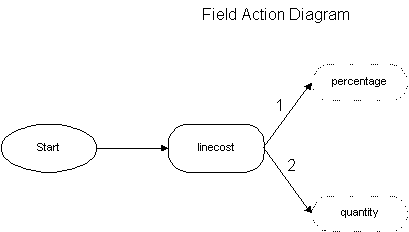psdi.app.invoice

Class FldInvoiceCostLineCost

• All Implemented Interfaces:
MboConstants, MboValueListener
Direct Known Subclasses:
FldInvoiceCostLineCostForUI

public class FldInvoiceCostLineCost
InvoiceCost.linecost validation class: Checks if the invoice cost line cost is not greater than the invoice line cost. Also when calculating and setting percentage field make sure all the invoice cost lines for that set totals to 100% when the sum of the linecost for the same is equal to the invoice line linecost. The sum of the invoice cost lines quantity should also equal to the invoiceline quantity.
Author:
Aman Thakur
• Constructor Detail

• FldInvoiceCostLineCost

public FldInvoiceCostLineCost(MboValue mbv)
• Method Detail

• action

public void action()
throws MXException,
java.rmi.RemoteException
Changing the invoice cost linecost changes the percentage and the quantity for that invoice cost. If the quantity on the invoice line is null only the percentage on the invoice cost is set. If the sum of the invoice cost lines linecost is equal to the invoice line linecost, the percentage of the current invoice cost should be calculated to a value so that the percentage total of the invoice cost lines is 100%. e.g If an invoice line has 3 invoice cost lines with a linecost of 1 each. The percentage of the first two lines is 33.33% each, but the percentage of the third line should be calculated to 33.34% so that the total adds to 100%. Also the sum of the invoice cost lines quantity should be equal to the invoice line quantity.Conditions:
1. If the total of linecost of the invoicecost lines is equal to the linecost on the corresponding invoiceline then round off the percentage on the last invoicecost line so that the sum oof the percentages on the invoicecost lines comes to 100 otherwise calculate the percentage using the formula ,percentage=(current InvoiceCostLineCost/invoiceLineLineCost) * 100
2. If the total of the linecost on the invoicecost lines is equal to the linecost on the corresponding invoiceline set the invoicecost quantity as the difference between the invoiceline quantity and the sum of the other invoicecost lines otherwise calculate quantity = invoiceLineQty * percentage of this invoicecost line/100
Specified by:
action in interface MboValueListener
Overrides: